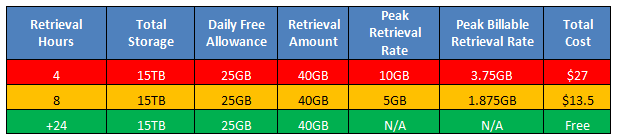Understanding AWS Glacier Retrieval Costs - Not as simple as you may have thought!

Published on
8,516 Points
2,116 Views
4 Endorsements
Approved
AWS Glacier is Amazons cheapest storage option and is their answer to a ‘Cold’ storage service.  Customers primarily use this service for archival purposes and storage of infrastructure backups.  Its unlimited storage potential and low storage cost makes it a popular storage choice.

What can be sometimes overlooked are the retrieval costs of your data, depending on how much you retrieve and over what time period can make a huge difference.  This article will cover these costs and help you understand the considerations of data retrieval.  Its important to note that AWS prices are exclusive of any GST or VAT chargeable (more information on these fees can be found here.)

Retrieval Costs

When you find yourself in a situation where you need to retrieve data from Glacier you need to understand some of the costs to ensure you can complete it in the most cost effective way.

You will probably be aware that AWS boasts a retrieval rate of \$0.01 per GB; however this is not as simple to calculate as it seems.

It’s important to note that you can retrieve up to 5% of your total AWS Glacier for free each month which is monitored on a pro rata basis daily.  Therefore if for example you have 15TB of data stored on Glacier you could retrieve 25GB a day for free (15TB * 5% (0.05) / 30(days) = 25GB).  25GB would be your daily free allowance.

Any data retrieved over this amount per day will be chargeable based upon other calculations which I will explain.  Before continuing with this explanation I want to describe some terms used in this retrieval pricing:

• Peak Retrieval Rate: This corresponds to your largest amount of data retrieved in an hour within a month.  AWS assumes that a retrieval job completes in 3-5 hours, but uses a figure of 4 hours for their calculations
• Peak Billable Retrieval rate:  This is reached by subtracting your daily free allowance from the Peak Retrieval Rate above

With this in mind, let’s assume you wanted to retrieve 40GB from the 15TB example above.

To do this we would need our Peak Retrieval Rate (PRR) which can be calculated by:  40GB/4 hours = 10GB per hour.  The PRR would be 10GB.

We then need to calculate our Peak Billable Retrieval Rate (PBRR). Using the original example we are allowed 25GB free a day which gives us:  25GB/4 hours = 6.25GB free per hour.  Therefore the PBRR would be:  10GB-6.25GB = 3.75GB per hour.

Now we have these figures we can calculate how much this would cost to retrieve all at once over those 4 hours.  This is done by multiplying our PBRR (3.75GB) by the retrieval fee which is as we know \$0.01/GB which is then multiplied by the number of hours in a month (720).  This gives us the end result of:  3.75GB * \$0.01 * 720 Hours = \$27

Therefore to retrieve 40GB of data in one retrieval period between 3-5 hours it would cost \$27 and not \$0.015 which you may have initially thought by 40GB-25GB = 15GB * 0.01\$ = \$0.015.

A great way to reduce these costs (if time permits) is to retrieve your data in multiple smaller blocks.  Let’s look at the same example but over 8 hours (assuming all completed within the same day), so 2 separate retrievals.

20GB would be requested from each retrieval meaning our PRR would be: 20GB/4 Hours = 5GB Per hour.

The PBRR is now calculated by the total number of hours the complete retrieval would take, so in this case 8 hours.  When doing this you need to adjust for your daily free allowance too with this figure, therefore 25GB/8 Hours = 3.125GB free per hour.  Therefore the PBRR would be: 5GB – 3.125GB = 1.875GB per hour.

Again, to find the end cost we use the formula of PBRR * \$0.01 * 720 Hours:

1.875GB * \$0.01 * 720 Hours = \$13.5

As you can see by splitting the retrieval of data into smaller blocks over a longer time period it has in this instance halved the price from \$27 to \$13.5.  With this in mind, if your data is not required all at once then I recommend you splitting your retrievals into smaller parts as you can potentially save considerable amounts of money.

One final note on this scenario, if you we to split this retrieval over a period longer than +24 hours then the download would be free due to the fact that we would be allowed 25GB free per day.My key note of this article is that before you start to use AWS Glacier ensure you have a full understanding of all costs involved.  Don’t just focus on the actual storage costs of the data (which is undeniably very cost effective), but what is often overlooked and can be more important, the costs of retrieval as they can be surprisingly high to some people.

4